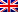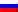# Bellman's algorithm

Contenus

## Bellman's algorithm

Bellman's algorithm is based on the following observation: if we know all the values of the edges (u, v) with u in a subgraph and v outside this subgraph. If we know all the shortest paths to u, then we can calculate d (v) = min {d (u) + weight (u, v)}. The Bellmann algorithm is a greedy dynamic programming algorithm (similar to a first bread search), it visits all the possible solutions.

Conditions.

• No cycle
• Arc only
• Finite number of vertices
• A source (and incidentally a target) defined

Bellman's algorithm takes as input a directed graph weighted by positive reals and a source vertex. This involves progressively building a subgraph in which the different vertices are classified in increasing order of their minimum distance from the starting vertex. The distance corresponds to the sum of the weights of the borrowed arcs.

Initially, we consider that the distances from each vertex to the starting vertex are infinite except for the starting vertex for which the distance is 0. The starting subgraph is the empty set.

During each iteration, we choose outside the subgraph a vertex such that all its successors are in the subgraph and we add it to the subgraph. Then, we update the distances of the neighboring vertices of the added one. The update takes place as follows: the new distance from the neighboring vertex is the minimum between the existing distance and that obtained by adding the weight of the arc between neighboring vertex and vertex added to the distance from the added vertex.

We continue in this way until the summits are exhausted (or until the arrival summit is selected).

For practical reasons, solving the Bellman algorithm only returns a vector containing the visited vertex, the list of predecessors (the vertices already validated) and the values of the shortest paths to all the other updated vertices. Which corresponds to a current row of the presented table.

Optimality

A vertex is validated if all of its predecessors are validated. That is to say, we know the shortest path of all its predecessors. In the initial state, we have validated the origin and we know the size 1 paths starting from the origin. The next vertex to validate therefore has a path with only validated vertices. By induction we deduce that we have validated the shortest path from the origin to this vertex.

```D  = 0;
for each vertex i ‚0 make C [i] = 0; D [i] = L [1, i];
for k = 1 year - 1 do
for each i successor of k do
if (D [k] + L [k, i] <D [i]) then D [i] = D [k] + L [k, i]; C [i] = k;
end
end
endFRFRENRUES